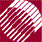ECE532 Biomedical Optics © 1998 Steven L. Jacques, Scott A. Prahl Oregon Graduate Institute

# Definition and units of absorption coefficient µa [cm-1]

Consider a chromophore idealized as a sphere with a particular geometrical size. Consider that this sphere blocks incident light and casts a shadow, which constitutes absorption. This description is of course an incorrect and schematicized version of the real situation. However, it does provide a simple concept which captures the essence of the absorption coefficient, the parameter we use to describe the effectiveness of absorption.

The size of the absorption shadow is called the effective cross-sectiona [cm2]) and can be smaller or larger than the geometrical size of the chromophore (A [cm2]), related by the proportionality constant called the absorption efficiency Qa [dimensionless]: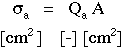The absorption coefficient µa [cm-1] describes a medium containing many chromophores at a concentration described as a volume densitya [cm3]. The absorption coefficient is essentially the cross-sectional area per unit volume of medium.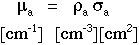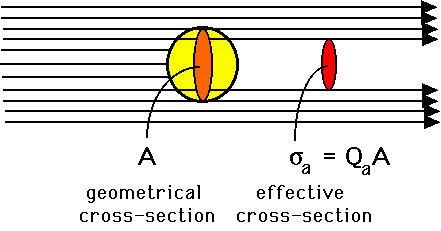Experimentally, the units [cm-1] for µa are inverse length, such that the product µaL is dimensionless, where L [cm] is a photon's pathlength of travel through the medium. The probability of survival (or transmission T) of the photon after a pathlength L is: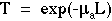This expression for survival holds true regardless of whether the photon path is a straight line or a highly tortuous path due to multiple scattering in an optically turbid medium.